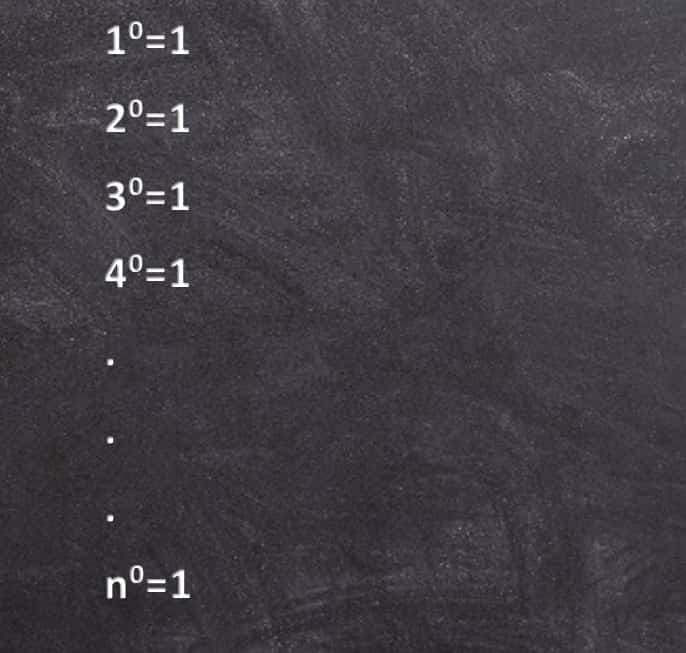# Why is any number raised to the 0 power equal to 1?

0Shares

## It is commonly taught that any number raised to the 0 power equal to 1 why is it so lets see,Any number raised to the 0 power equal to 1, can be explained with these methods,

 1) Let’s first look at an example. Let’s look at the list of numbers ``` 3^1, 3^2, 3^3, 3^4, .... Finding the actual values, we get 3, 9, 27, 81, .... ``` So what is the pattern in the bottom sequence? Well, every time you move to the right in the list you multiply by 3, and every time you move to the left in the list you divide by 3. So we could take the bottom sequence and keep going to the left and dividing by 3, and we’d have the sequence that looks like this: ```..., 3^-3, 3^-2, 3^-1, 3^0, 3^1, 3^2, 3^3, 3^4, .... ..., 1/27, 1/9, 1/3, 1, 3, 9, 27, 81, .... ``` So now we know what all the powers of 3 are! Actually, we just did the integer powers of 3. But that’s probably enough for now. 2) While the above argument might help convince your intuitive side that any number to the zero power is 1, the following argument is a little more rigorous.This proof uses the laws of exponents. One of the laws of exponents is: ``` n^x --- = n^(x-y) n^y ``` for all n, x, and y. So for example, ``` 3^4 --- = 3^(4-2) = 3^2 3^2 3^4 --- = 3^(4-3) = 3^1 3^3 ``` Now suppose we have the fraction: ``` 3^4 --- 3^4 ``` This fraction equals 1, because the numerator and the denominator are the same. If we apply the law of exponents, we get: ``` 3^4 1 = --- = 3^(4-4) = 3^0 3^4 ``` So 3^0 = 1.We can plug in any in number in the place of three, and that number raised to the zero power will still be 1. In fact, the whole proof works if we just plug in x for 3: ``` x^4 x^0 = x^(4-4) = --- = 1 x^4 ``` 3) Here’s another explanation from Aldo Daniel Completa, a contributor from Argentina:In the FAQ there is an explanation about ” n^0 (any number to zero power) .” I think another is this: Let’s begin with examples. ``` Ex.1: 5^3 / 5^2 = 125 / 25 = 5 5^3 / 5^2 = 5 ^(3-2) = 5^1 = 5 Ex.2: 5^3 / 5^3 = 125 / 125 = 1 5^3 / 5^3 = 5 ^(3-3) = 5^0 = ``` … and the result must be 1. So 5^0 =1.The rule is: x^b / x^c = x^(b-c). In order to generalize this rule for the case b=c, it must be defined that x^0 = 1 (x is any number different from 0). In mathematics, usefulness and consistency are very important. This convention allows us to extend definitions of power that would otherwise require treating 0 as a special case. This method can also explain the definition: x^(-b) = 1 / x^b. ``` Ex. 5^2 / 5^4 = 25 / 625 = 1 / 5^2 5^2 / 5^4 = 5 ^(2-4) = 5^(-2)```

Source: http://mathforum.org/

## One Thought to “Why is any number raised to the 0 power equal to 1?”

1.Anonymous

Thank You…